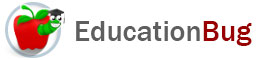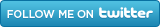PUBLIC SCHOOLS PRIVATE SCHOOLS SCHOOL DISTRICTS COLLEGES PUBLIC LIBRARIES JOBS BLOG RESOURCES
Career Education
Education Choices
Education Costs
Education History and General Information
Education Issues
Education Resources
Family Involvement in Education
Students w Special Needs
Subject Information and Homework Help
Teaching and Learning
Testing
By Types of Schools
By Specific Audiences
GeneralSubtraction

Subtraction is one of the main four operations of mathematics you can use to solve a problem, or answer a question. This article has information on forms of subtraction, regrouping or borrowing, and subtracting negative numbers.

Youâ€™re in the store with a ten-dollar bill, and have already chosen one item, when you see a two-for sale on something else you werenâ€™t planning to get. Can you afford it? And what is your bank balance anyway? Questions like these are often addressed by using subtraction, one of the four basic arithmetic operations, along with addition, multiplication, and division.

Subtraction is the inverse of addition, and sometimes itâ€™s easy to substitute addition for subtraction. For example, in the store example, you could either:

a) subtract the price of your first item from \$10 and see if what you have left is greater than or equal to the cost of the two-for special (donâ€™t forget tax!); or

b) you could add the price of the first item to the price of the two-for special and see if the result is less than or equal to \$10.

But sometimes, it can be easier to just subtract. For more on this topic, keep reading.

The Nomenclature of Subtraction

Subtraction has three elements: the number you are starting with, the number youâ€™re subtracting, and the result. Although the mathematical names for these elements are not frequently used, it can be useful to know them so that if they do come up, the terms wonâ€™t be completely foreign to you. Here they are:

Subtraction

 c - b = a minuend minus sign subtrahend equals sign difference

Where do the two very unusual names come from? Minuend comes from the Latin minuendum, which means â€œthe thing to be diminishedâ€ from the verb minuere, which means â€œto lessen.â€ Subtrahend comes from the Latin subtrahendum, which is a form of the verb subtrahere, meaning â€œto subtract.â€

Forms of Subtraction

Subtraction problems may appear in the form of word problems or be expressed in mathematical symbols either horizontally or vertically. The horizontal form is like the chart above, just without the chart:

c - b = a

The vertical form aligns the places (see the article â€œDecimalsâ€ for a discussion of the decimal notation system), which can make subtraction easier to think about when large numbers of digit are involved.

c

- b

a

Compare the two forms with a problem like the following, and youâ€™ll see what I mean:

713.72859 - 28.113 =

713.72859

- 28.113

Regrouping or Borrowing

Regrouping or borrowing is a process that redistributes the value of a number in terms of the decimal places that are being used to represent it, taking value from places that appear farther left and moving the value farther right. This is used by some subtraction methods in the decimal system when we subtract on paper place-by-place, rather than all at once on a calculator.

When Subtraction Involves Negative Numbers

If a larger positive number is subtracted from a smaller positive number, the result will be a negative number. Some people have a difficult time conceiving of negative numbers. Sometimes thinking about temperature and weather thermometers can be helpful because this is an area in which the use of negative numbers is most familiar.. Number lines can also help make the working with negative numbers easier. For more information, see the article â€œNumber Lines.â€

Addition and subtraction have some differences in how they operate. In addition, you can switch the order of the addends (the numbers being added) without affecting the sum. But it is not possible to switch the minuend and the subtrahend without changing the difference.

5 + 4 = 4 + 5

5 - 4 â‰  4 - 5

Another way of saying this is that addition is commutative, but subtraction is not.

Also, in addition, one can treat multiple addends in any order without affecting the sum, while the same is not true of subtraction.

(7+2) + 1 = 7 + (2 + 1)

(7-2) - 1 = 7 - (2 - 1)

Another way of saying that is that addition is associative, but subtraction is not.

Because of these two features of addition that are not shared by subtraction, it can be useful to reconceive subtraction problems as addition problems by using negative numbers:

7 - 3 â‰  7 + (-3)

The answer is the same, but while one is solving the problem, the associative and commutative properties can be used, which in more complex examples than the one Iâ€™ve given above, can be extremely useful.

Related Articles
Number Lines Decimals